TAG: Integrals
November 1, 2010–

Wolfram|Alpha is still young and constantly improving. One of the biggest hurdles that our developers are constantly faced with is how to correctly interpret the meaning of general user inputs like “How do I factor an equation?”

Wolfram|Alpha is great at calculating answers that have specific inputs, but when general concepts are given (like “factoring”, for example), it becomes a bit tricky. How would one calculate a concept like that? Let us look at a specific example—Wolfram|Alpha can easily calculate integrals, as long as you ask it to integrate an explicit function. But what happens when you simply ask Wolfram|Alpha to “integrate”? Before, had you given this input, a number of examples using the word “integrate” would have been generated to show how to properly ask Wolfram|Alpha to calculate an integral. But now, when you enter a generic term or question related to a specific math function or formula, it provides a simple query-specific calculator.

For example, given the query “Show me how to integrate”, the following results appear:

Notice that new input fields appear (as well as optional ones in case the integral is to be calculated within a range, and/or with multiple variables). Similarly, notice how the input fields differ depending on the query: More »

February 5, 2010–

Version 1.1 of the Wolfram|Alpha App for the iPhone & iPod is now available in the App Store. The new version includes a number of new features that continue to improve the app’s unique mobile Wolfram|Alpha experience. Perhaps its most iconic feature, the specialized keyboards that greet you when you first open the Wolfram|Alpha App, have been painstakingly constructed to ease the burden of entering queries, whether you’re converting from pounds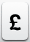to euros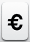or computing a numerical value for the Weierstrass p-function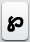. Our goal in creating these keyboards was to form families of characters that naturally occur together both in common use and in traditional mathematical applications. We also wanted mathematical expressions to look and feel natural to enhance usability and understanding. Version 1.1 has four specialized keyboards: the default keyboard, the “math” keyboard accessed by the right-shift key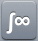, the “Greek” keyboard accessed by one press of the left-shift key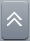, and the “symbol” keyboard accessed by a second press of the left-shift key.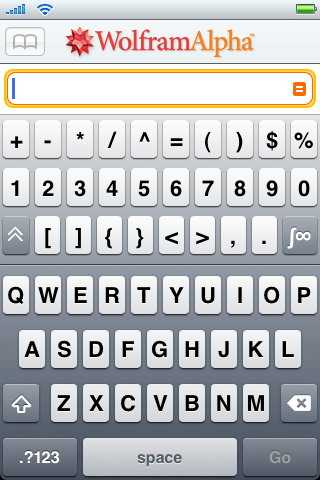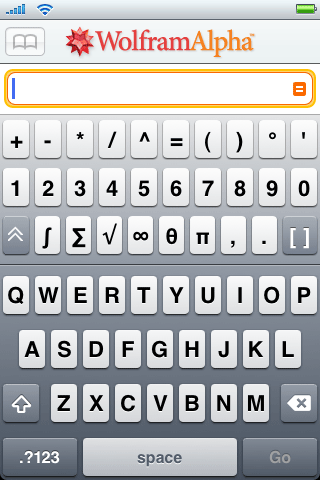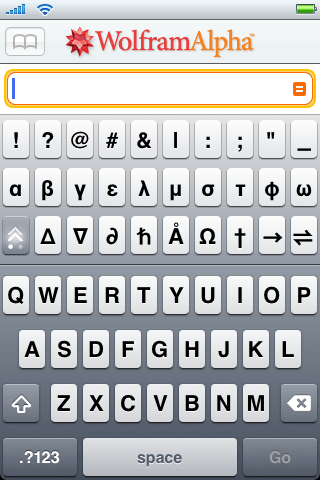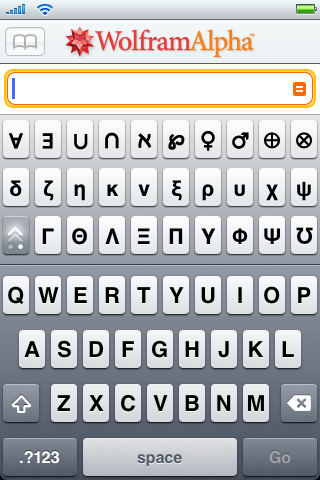To determine the optimal keyboard layout, we scoured Wolfram|Alpha’s server logs for the most commonly entered phrases that have characters with meaning in Wolfram|Alpha. Given that Wolfram|Alpha is built on Mathematica, one of its core strengths is advanced mathematics. True to form most of the commonly typed characters are related to math. For example, you would generally type the word “integrate” to compute an integral on the Wolfram|Alpha website. In the Wolfram|Alpha App you could simply type the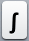key on the math keyboard. The same is true for other symbols common in math, such as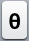and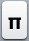. Specifying geometric shapes, such as a triangle, is straightforward as well.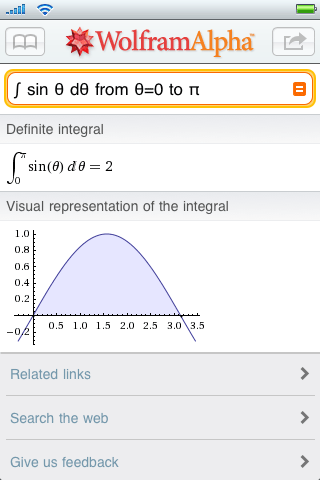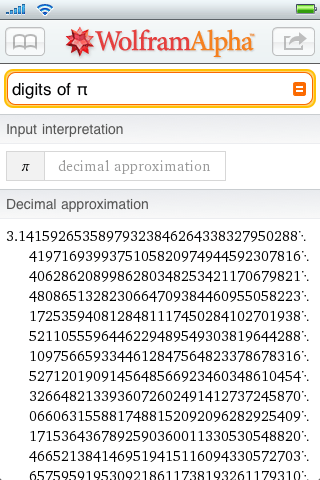More »

December 1, 2009–

## July 17, 2019 Update: Step-by-step solutions has been updated! Learn more.

Have you ever given up working on a math problem because you couldn’t figure out the next step? Wolfram|Alpha can guide you step by step through the process of solving many mathematical problems, from solving a simple quadratic equation to taking the integral of a complex function.

When trying to find the roots of 3x2+x–7=4x, Wolfram|Alpha can break down the steps for you if you click the “Show steps” button in the Result pod.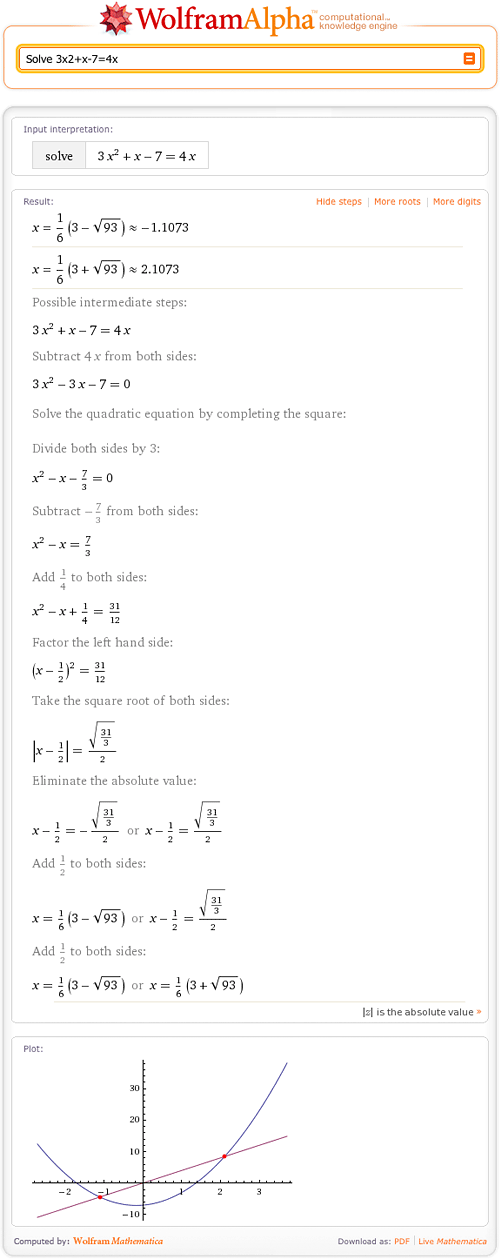As you can see, Wolfram|Alpha can find the roots of quadratic equations. Wolfram|Alpha shows how to solve this equation by completing the square and then solving for x. Of course, there are other ways to solve this problem! More »